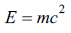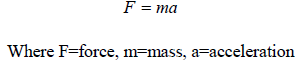All submissions of the EM system will be redirected to Online Manuscript Submission System. Authors are requested to submit articles directly to Online Manuscript Submission System of respective journal.

# Original Article

, Volume: 11( 4) DOI: 10.37532/2320-6756.2023.11 (4).338

## A new phenomenology of force and energy

*Correspondence:
Giustino Travaglini
C.da Quarto da Capo n°14 i.2, 66043, Casoli (CH), Italy
E-mail: travaglinigiustino@gmail.com

Received date: 27-March-2023, Manuscript No. tspa-23-93109; Editor assigned: 4-April-2023, Pre-QC No. tspa-23-93109 (PQ); Reviewed: 11- April-2023, QC No. tspa-23-93109 (Q); Revised: 16-April-2023, Manuscript No. tspa-23-93109 (R); Published: 27-April-2023, DOI. 10.37532/2320-6756.2023.11 (4).338

Citation: Travaglini G. A New Phenomenology of Force and Energy. J. Phys. Astron.2023; 11(4):338.

### Abstract

Until now in modern physics energy and force have been considered two separate and distinct entities, the various forces of nature have not been linked into a single, coherent theory, and general relativity shows inconsistencies with some mechanical phenomena quantum. The purpose of this article is to show that energy and force are a single entity which I will refer to as "energy force" furthermore general relativity can harmonize with quantum mechanics in a single theory but needs to be expanded. To arrive at this result, I used recent studies carried out on some phenomena of quantum mechanics, in particular on the existence, nature and characteristics of "quantum entanglement". The results obtained from the observation and study of "quantum entanglement" show that energy and force are one and that light is to be considered not as an entity that moves with a constant speed but as a accelerating entity which, under certain circumstances, can exceed the 300,000 km/s barrier.

### Keywords

Harmonize; Energy

### Introduction

Entanglement or quantum correlation is a quantum phenomenon, not reducible to classical mechanics, for which, in the condition, foreseen by the superposition principle of quantum mechanics, in which two or more physical systems (typically two particles) form a system whose quantum state is represented by a combination of their individual states, the measurement of an observable of a system (subsystem) also simultaneously determines the value of the same observable of the others. Since the state of quantum superposition is independent of a spatial separation of such systems (subsystems), entanglement implies in a counterintuitive way the presence of distant correlations between them and, consequently, the non-local character of the physical reality. According to quantum mechanics it is possible to create a system consisting of two particles characterized by certain global values of some observables. This implies that the value of one of these measured on a single particle (a typical example is spin) instantly influences the corresponding value of the other, which will be such as to maintain the initial global value, according to the conservation law. This remains true even in the experimentally possible case that the two particles are spaced apart, without any spatial limit. It should be noted that the measurement process relating to the single particle is subject to the quantum rules of probability. In practice, two particles can be obtained which, according to theory, should possess this characteristic, by making them interact appropriately or by acquiring them from a natural process that originates in the same instant (for example a single radioactive decay), so that they are described by a state defined global quantum, while individually maintaining an indefinite character until a measurement is performed. I will not dwell on the mathematical formalism as many studies carried out with photons are already known, neutrinos and electrons have demonstrated the existence of quantum entanglement [1-3].

### The Energy Force

The existence of quantum entanglement is indisputable however the phenomenon does not agree with the theory of relativity which, among its pillars, has the constant of the speed of light at about 300,000 km/s. We know that the formula for Energy is:Where E=energy, m=mass, c=constant (speed of light in vacuum).The constancy of the speed of light in a vacuum does not agree with the property of simultaneity present in quantum entanglement. Postulating that light is an accelerating entity and not an entity moving at a constant speed resolves this inconsistency. Called “c t” the way of the light speed in one second, set " ct = 3 × 108 [m]" that become a constant with the unit [m] and rewrite the energy formula:Where E=energy, m=mass, a=acceleration (acceleration of light), ct = 3 × 108 [m]

Now the simultaneity present in the phenomenon of quantum entanglement is explained as light exceeds the speed of 300,000 km/s remaining the fastest body existing in nature.

If it is true that:You get:Looking at the new Energy formula:We realize that the Energy formula is identical to that of the Force where:Consequently we have:Since m=m then:Therefore we get:This means that by expanding the theory of general relativity and considering light as an accelerating entity it is possible to conclude that force is equal to energy, therefore force and energy are a single entity which I will here call "energy force".

### Result

• There is an equivalence between energy and force which from now on will be considered as a single entity called "energy force";

• The phenomenology of energy- force confirms the correctness of the general and restricted theory of relativity which, however, are limited because they describe a particular case of the motion of light, i.e. the one in which it moves at a constant speed, not taking into account the more general case in which light is an accelerating entity;

• Quantum entanglement represents an empirical demonstration of light as an accelerating entity and not as an entity capable of moving only with constant speed;

• Light is the fastest-moving entity that exists in nature, when it travels in a vacuum it does so with a constant speed of 300,000 km/s however it is able to exceed the threshold of 300,000 km/ s under certain circumstances such as for example in the case of quantum entanglement

### Acknowledgement

Thanks to Wolfgang Sturm for suggesting the use of "ct" as a constant with the unit [m].

### References

Welcome Message

Select your language of interest to view the total content in your interested language

###### Citations : 272

Journal of Physics & Astronomy received 272 citations as per Google Scholar report

#### Indexed In

• Open J Gate
• China National Knowledge Infrastructure (CNKI)
• Cosmos IF
• Directory of Research Journal Indexing (DRJI)
• MIAR
• Secret Search Engine Labs
• Euro Pub
• clarivate-web-of-science-logo-vector
• ICMJE

View More

### 9th International Conference on Physics

Edinburgh, Scotland

### 6th International Conference on Astronomy, Astrophysics and Space Science

Budapest, Hungary

Berlin, Germany

### 9th International Conference on Physics

Edinburgh, Scotland

### 6th International Conference on Astronomy, Astrophysics and Space Science

Budapest, Hungary

### 10th International Conference on Theoretical and Applied Physics